Note

This page is a reference documentation. It only explains the function signature, and not how to use it. Please refer to the user guide for the big picture.

# nilearn.plotting.plot_matrix#

nilearn.plotting.plot_matrix(mat, title=None, labels=None, figure=None, axes=None, colorbar=True, cmap=<matplotlib.colors.LinearSegmentedColormap object>, tri='full', auto_fit=True, grid=False, reorder=False, **kwargs)[source]#

Plot the given matrix.

Parameters:
mat

Matrix to be plotted.

title`str`, or None, default=None

The title displayed on the figure.

labels`list`, or `numpy.ndarray` of `str`, or False, or None, optional

The label of each row and column. Needs to be the same length as rows/columns of mat. If False, None, or an empty list, no labels are plotted.

figure`matplotlib.figure.Figure`, figsize `tuple`, or None, optional

Sets the figure used. This argument can be either an existing figure, or a pair (width, height) that gives the size of a newly-created figure.

Note

Specifying both axes and figure is not allowed.

axesNone or `matplotlib.axes.Axes`, optional

Axes instance to be plotted on. Creates a new one if None.

Note

Specifying both axes and figure is not allowed.

colorbar`bool`, optional

If True, display a colorbar on the right of the plots. Default=True.

cmap`matplotlib.colors.Colormap`, or `str`, optional

The colormap to use. Either a string which is a name of a matplotlib colormap, or a matplotlib colormap object. Default=`plt.cm.RdBu_r`.

tri{‘full’, ‘lower’, ‘diag’}, optional

Which triangular part of the matrix to plot:

• ‘lower’: Plot the lower part

• ‘diag’: Plot the lower part with the diagonal

• ‘full’: Plot the full matrix

Default=’full’.

auto_fit`bool`, optional

If auto_fit is True, the axes are dimensioned to give room for the labels. This assumes that the labels are resting against the bottom and left edges of the figure. Default=True.

gridcolor or False, optional

If not False, a grid is plotted to separate rows and columns using the given color. Default=False.

reorder`bool` or {‘single’, ‘complete’, ‘average’}, optional

If not False, reorders the matrix into blocks of clusters. Accepted linkage options for the clustering are ‘single’, ‘complete’, and ‘average’. True defaults to average linkage. Default=False.

Note

This option is only available with SciPy >= 1.0.0.

New in version 0.4.1.

kwargsextra keyword arguments, optional

Extra keyword arguments are sent to pylab.imshow.

Returns:
display`matplotlib.axes.Axes`

Axes image.

## Examples using `nilearn.plotting.plot_matrix`#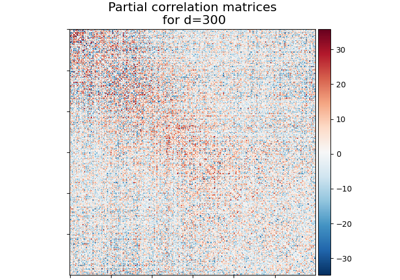Visualizing Megatrawls Network Matrices from Human Connectome Project

Visualizing Megatrawls Network Matrices from Human Connectome ProjectDecoding with FREM: face vs house vs chair object recognition

Decoding with FREM: face vs house vs chair object recognitionThe haxby dataset: different multi-class strategies

The haxby dataset: different multi-class strategies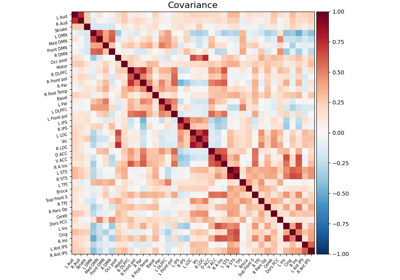Computing a connectome with sparse inverse covariance

Computing a connectome with sparse inverse covarianceExtracting signals of a probabilistic atlas of functional regions

Extracting signals of a probabilistic atlas of functional regions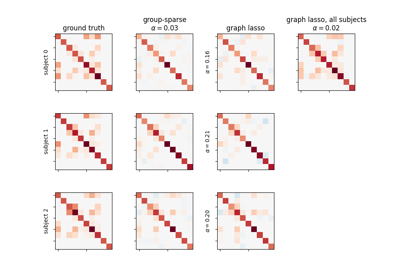Connectivity structure estimation on simulated data

Connectivity structure estimation on simulated dataGroup Sparse inverse covariance for multi-subject connectome

Group Sparse inverse covariance for multi-subject connectome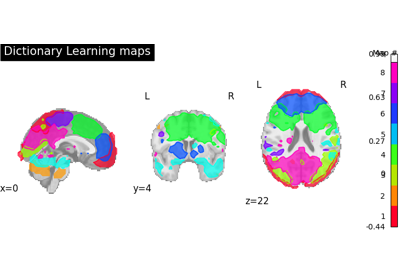Regions extraction using dictionary learning and functional connectomes

Regions extraction using dictionary learning and functional connectomes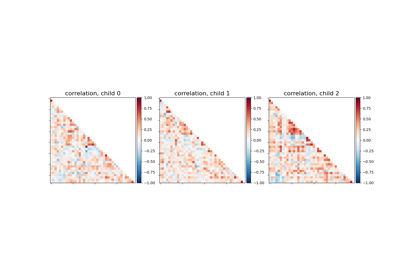Classification of age groups using functional connectivity

Classification of age groups using functional connectivity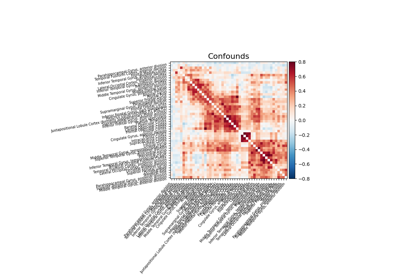Extracting signals from a brain parcellation

Extracting signals from a brain parcellation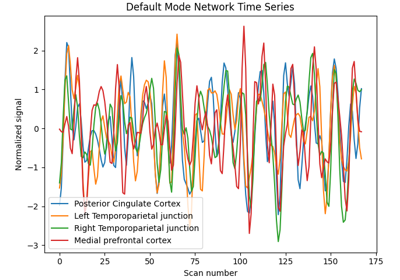Extract signals on spheres and plot a connectome

Extract signals on spheres and plot a connectome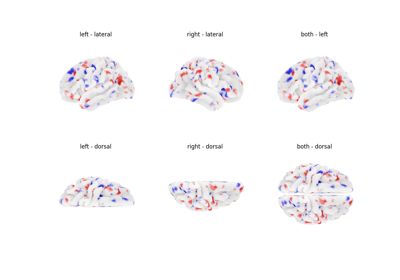A short demo of the surface images & maskers

A short demo of the surface images & maskers